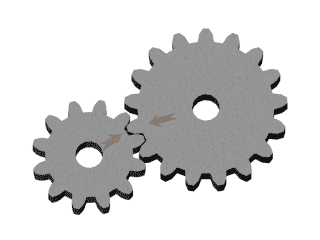# How Can We Help?

• Articles coming soon
• Articles coming soon
• Articles coming soon
• Articles coming soon
• Articles coming soon
• Articles coming soon
• Articles coming soon
• Articles coming soon
• Articles coming soon
• Articles coming soon
• Articles coming soon
• Articles coming soon
• Articles coming soon
• Articles coming soon
• Articles coming soon

# Rate Of Change

You are here:
• Rate Of Change

Calculus is branch of Mathematics which studies the continuous change. What we are going to learn in this article is to understand the core concept of rate of change and will intuitively derive the equation below by ourself.Let’s start with an example, suppose we have a pair of gears aligned in a specific configuration as shown below.Left Gear is Input Gear and Right Gear is Output Gear

Suppose, if we rotate input gear byamount then we can clearly see that the output gear will rotate in opposite direction as input gear and letbe the amount of rotation of output gear. We can observe that the amount of rotation of output gear depends on the amount of rotation of input gear. So, if we ask, What will be the change in the amount of rotation of output gear if we change the rotation of input gear?

To answer this, we know that the rotation of output gear depends on input gear and we can say that the amount of change in output gear will be some multiple ofi,e;. Let’s understand this first, we can write. For example, if we rotate the input gear by 2 units and if the output gear rotates 4 units (because the input gear is rotated), we can say that the change in output is 2 times the change in input, how do we get that? Just by dividing the output change by the input change. Finally, we can say that from the example if the change in input isthen the output will be changed byor we can say that the rate of change is 2 units.

Now, let’s assume a functionwhich is continuous and well defined between an interval ofandwhere,.

If we inputthen we are getting, but now let’s increase or change the value ofbythen our input will beand onwe will get, Let’s see that in figure below:

Now if we again ask, by what amount the functionwill change if we change the input by? From above we know that. So, let’s calculate each part, firstsimilarly,. Now, let’s find rate of change of function:If(), then we can write:So, here we can conclude this article on Rate Of Change, in coming articles we will see that how this simple concept is the foundation of all the modern learning algorithms used in deep learning.